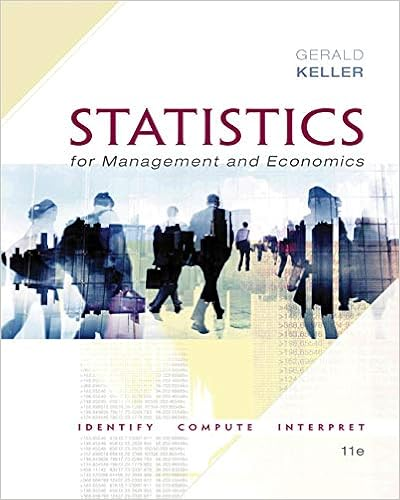# Are you ready for the job tasks q1 first assume fleet

• Notes
• 43
• 25% (4) 1 out of 4 people found this document helpful

This preview shows page 39 - 41 out of 43 pages.

##### We have textbook solutions for you!
The document you are viewing contains questions related to this textbook.The document you are viewing contains questions related to this textbook.
Chapter 22 / Exercise 22.8
Statistics for Management and Economics + XLSTAT Bind-in
KellerExpert Verified
Are you ready for the job? Tasks: Q1. First assume fleet size Q = 1500 . Consider a static pricing strategy: p 1 p 1 1 = p 1 2 = p 1 3 = p 1 4 = \$40 , i.e., set the same price \$40 for four weeks. Simulate the operation of Tampa branch for n = 10 3 (sample size) months (sample path with 4 observations). Compute the expected fill rate E [ f ] , total expect monthly profit V Q ( p 1 ) , and its 95% confidence interval. Q2. Assume Q = 1500 . Repeat question 1 for p 1 ∈ { 45 , 50 , . . . , 70 } . Graph V Q ( p 1 ) against p 1 and find the optimal price p 1 that maximizes the total profit V Q = max p 1 V Q ( p 1 ) . Q3. Now change Q = 2000 , repeat questions 1 and 2. Find the optimal price p 1 and total profit V Q for fleet size Q = 2000 . Q4. Repeat questions 1 and 2, for Q = { 2500 , 3000 , . . . , 4000 } . For each Q , find the optimal associated price p 1 and the optimal total profit V Q . Graph V Q against Q . Find the optimal fleet size Q . Bonus (optional): Now consider a dynamic pricing strategy. Suppose you may set the price p 1 t for each week differently, where p 1 t ∈ { 40 , 45 , . . . , 70 } . By the similar approach in questions 1- 3, find the optimal price path ( p 1 1 , p 1 2 , p 1 3 , p 1 4 ) for each fleet size Q ∈ { 1500 , 2000 , . . . , 4000 } and 39
##### We have textbook solutions for you!
The document you are viewing contains questions related to this textbook.The document you are viewing contains questions related to this textbook.
Chapter 22 / Exercise 22.8
Statistics for Management and Economics + XLSTAT Bind-in
KellerExpert Verified
its associated optimal profit V Q . Graph V Q against Q . Find the optimal Q . Compare the total profit under the dynamic pricing strategy with that under the static pricing strategy. Which one performs better? By how much? Why? 7.3 Revenue Management An airline offers two fare classes for coach seats on a particular flight: full-fare class at \$440/ticket and economy class at \$218/ticket. There are x = 230 coach seats on the aircraft. Demand for full-fare seats has a mean of 43, a standard deviation of 8, and the following empirical distribution (it is not normal distribution, so we need to use inverse transform method to generate random demand). Economy-class customers must buy their tickets three weeks in advance, and these tickets are expected to sell out. For given capacity x , let y ( x ) be the protection level, b ( x ) = x - y ( x ) the booking limit for low-fare seats, V ( x ) the maximal expected profit, and p ( x ) = V ( x ) - V ( x - 1) the marginal value of x th seat. Assume sample size N = 10 3 . a) Rationing: For capacity x = 230 , find V (230) , y (230) , and b (230) via the following two meth- ods: analytical method (marginal value argument), and brute force simulation defined below. 6 b) For capacity x = 100 , find the profit V (100) and protection level y (100) . Do V ( x ) and y ( x ) change? Why or why not? c) Dynamic pricing: Now compute V ( x ) and p ( x ) for each x = 1 , 2 , . . . , 230 . Plot V ( x ) and p ( x ) against capacity x . Base one these results, if you were the manager, what price should you charge for each seat? Can you explain why the ticket price typically increases when approaching the departure date? d) Suppose that unsold seats may sometimes be sold at the last minute at a very reduced rate (sim- ilar to USAirways’ “Esaver” for last-minute travel). What effect will this have on the protection level calculated in (a)?
•••